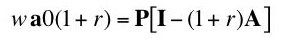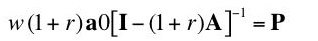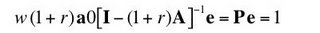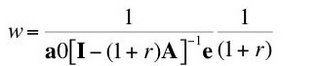## Wednesday, August 23, 2006

### Marginal Productivity Not A Theory Of Distribution (Part 3)

5.0 The "Factor Price Frontier"
This post builds on an analysis of marginal productivity in an economic model.

One can rewrite Equation 17 as Equation 20:(20)

Or:(21)

The matrix inverse exists for all r between zero and some maximum, inclusive, for each technique if both goods are basic and the economy is viable.

Let e be a column vector denoting the numeraire. Post-multiplying, one obtains:(22)

Hence, the wage-rate of interest curve for a given technique is:(23)

This is a downward sloping curve in w-r space, and it cuts both axis.

One can plot the wage-rate of interest curve for each technique. A technique is a set of coefficients of production, a0 and A. The coefficients of production a01, a11, a21, for the wheat industry lie on the unit-isoquant production function for wheat. Likewise, the coefficients of production a02, a12, a22, for the corn industry lie on the unit-isoquant production function for corn. Given a common numeraire used in plotting each curve, these curves fall in the same space, as in this example.

Consider the frontier formed by the outer envelope of all these curves. That is, for a given interest rate, the wage on the factor price frontier is the maximum wage from out of the wages on all the wage-interest rate curves at that rate of interest. Similarly, for a given wage, the factor price frontier shows the maximum rate of interest. And the coefficients of production used in constructing wage-rate of interest curves on the frontier for, say, a given rate of interest can be chosen by competitive profit-maximizing (or cost-minimizing) firms paying the given rate of interest and the equilibrium wage thereby determined.

The coefficients of production found in this analysis of the factor price frontier are the same as those that arise in the marginal productivity analysis. When the production functions are well-behaved and continuously differentiable, no point on the frontier is a switch point, and the optimizing coefficients of production vary continuously with the interest rate. Since the two analyses, of the factor price frontier and of marginal productivity, are equivalent, the wage is equal to the present value of the marginal product of labor for every point on the frontier.

The analyses of the factor price frontier, however, can be used to determine the cost-minimizing coefficients of production when the industry-specific production functions are not smooth. That is, the factor price frontier can be constructed when the set of possible coefficients of production is discrete. In this sense, the analysis of the factor price frontier, inasmuch as it applies to both continuously differentiable production functions and a discrete model of production, is more general than an analysis confined to cases where derivatives exist. (This is not a radical conclusion; post (Second World) war mainstream economists do not confine themselves to calculus-based results; they have long used topological arguments.)